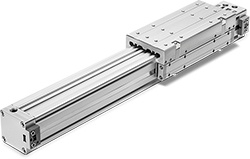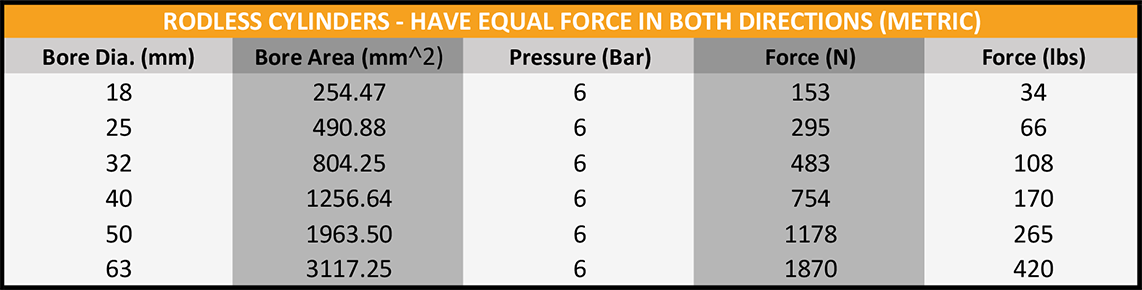715-426-2000

Solutions in Motion™When choosing actuators for industrial applications, you have to know the amount of force that will be required to perform the job at hand. Cylinders can move, slow, or hold a load -- rapidly and repeatedly, if needed. The force required depends on the load itself – its weight and movement angle, among other factors. An air cylinder force calculator can help you spec exactly the right actuator for the job. But wherever would you find one of those?

How the Pneumatic Cylinder Force Calculator Works

You could do the math by hand, but why bother? We have created three calculation charts, each of which corresponds to theyou expect to use. W.C. Branham makes cylinders in both metric and imperial sizes, so there are separate charts for each.

The formula to determine cylinder output force is: F = A x P

Where:

• F = cylinder force (lbs)
• A = cylinder bore area (in^2)
• P = Pressure applied (lbs/sq-in)

Note that these calculations provide theoretical force output values. They do not consider the loss of force due to the cylinder’s internal friction. The typical break-away pressure needed to overcome force lost due to friction is 5-8 PSI. Your goal is to achieve a force that is high enough to give you a margin of error, without overdoing it. Too much force and you will end up with broken components.

Rodless Cylinder Force Calculator — Equal Force in Both Directions

The table below helps to determine the general force required for rodless cylinders that will need to apply equal force in both directions. These double-acting cylinders are good choices for a wide variety of back-and-forth or up-and-down sequential movements such as lifting, lowering, clamping, sorting, knifing, or punching.Rod Cylinder Force Calculator — Unequal Force

Use either the imperial or metric table below to determine the general force required for rod cylinders that apply unequal force in each direction. These single-action cylinders either extend or retract, using gravity or spring action to return. They are good choices for actions such as clamping, ejecting, or activating flaps or levers.Cable Cylinder Force Calculator — Equal Force in Both Directions

Use the table below to determine the general force required for cable cylinders, which apply equal force in both directions.Get a Customizable Pneumatic Cylinder Force Calculator

Along with downloading the basic calculation tables illustrated above, you can also use this to tailor calculations for each project. As always, if you have any questions we're happy to guide you in the right direction. No question is too small. Contact our team of experts today!Topics: Pneumatic Actuators, Cylinders